Journal topic
J. Sens. Sens. Syst., 9, 117–125, 2020
https://doi.org/10.5194/jsss-9-117-2020J. Sens. Sens. Syst., 9, 117–125, 2020
https://doi.org/10.5194/jsss-9-117-2020Regular research article 26 Mar 2020

Regular research article | 26 Mar 2020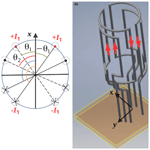# Homogenous nuclear magnetic resonance probe using the space harmonics suppression method

Homogenous nuclear magnetic resonance probe using the space harmonics suppression method
Pauline de Pellegars1,2, Liu Pan1, Rahima Sidi-Boulenouar1,3, Eric Nativel4, Michel Zanca1,5, Eric Alibert1, Sébastien Rousset1, Maida Cardoso1, Jean-Luc Verdeil3, Nadia Bertin6, Christophe Goze-Bac1, Julien Muller2, Rémy Schimpf2, and Christophe Coillot1 Pauline de Pellegars et al.
• 1Laboratoire Charles Coulomb (L2C-UMR5221), CNRS, University of Montpellier, Place EugeneBataillon, Montpellier, France
• 2RSD, 13 rue Vauban, 67450 Mundolsheim, France
• 3Centre de Cooperation Internationale en Recherche Agronomique pour le Développement (CIRAD), UMR AGAP, Montpellier, France
• 4Institut d'Electronique et des Systèmes (IES-UMR5214), University of Montpellier, Campus Saint-Priest, 34095 Montpellier, France
• 5Nuclear medicine, CMC Gui de Chauliac, University Hospital Montpellier, 34095 Montpellier, France
• 6Institut National de la Recherche Agronomique (INRA), PSH (UR 1115), Avignon, France

Correspondence: Christophe Coillot (christophe.coillot@umontpellier.fr)

Abstract

Nuclear magnetic resonance imaging (MRI) has became an unavoidable medical tool in spite of its poor sensitivity. This fact motivates the efforts to enhance the nuclear magnetic resonance (NMR) probe performance. Thus, the nuclear spin excitation and detection, classically performed using radio-frequency coils, are required to be highly sensitive and homogeneous. The space harmonics suppression (SHS) method, already demonstrated to construct coil producing homogenous static magnetic field, is used in this work to design radio-frequency coils. The SHS method is used to determine the distribution of the electrical conductive wires which are organized in a saddle-coil-like configuration. The theoretical study of these SHS coils allows one to expect an enhancement of the signal-to-noise ratio with respect to saddle coil. Coils prototypes were constructed and tested to measure 1H NMR signal at a low magnetic field (8 mT) and perform MRI acquired at a high magnetic field (3 T). The signal-to-noise ratios of these SHS coils are compared to the one of saddle coil and birdcage (in the 3 T case) of the same size under the same pulse sequence conditions demonstrating the performance enhancement allowed by the SHS coils.

1 Introduction

When nuclei having a nuclear spin are immersed in a magnetic field (B0), an aligned magnetization (M), resulting from the balance between parallel and anti-parallel magnetic moments, occurs. Besides this net magnetization, the nuclear spins precess at the Larmor angular frequency: ${\mathit{\omega }}_{\mathrm{0}}=-\mathit{\gamma }{B}_{\mathrm{0}}$, where γ is the gyromagnetic ratio of a given nucleus. The nuclear spin is disturbed by applying a weak radio-frequency magnetic field B1 at the Larmor frequency. Thus, the magnetization (M) is tilted, offering the possibility to detect the electromagnetic field associated with the precession and return to the thermal equilibrium (). The excitation and detection of the nuclear spins are usually performed using radio-frequency (RF) coils, also referred to as NMR/MRI (nuclear magnetic resonance/magnetic resonance imaging) probes. High signal-to-noise ratio (SNR) and homogeneity remain the main desirable features of these NMR/MRI probes. Research in this area remains active, and various MRI probe configurations have been proposed to address this need (): solenoid, saddle coil, birdcage, Bolinger coil, etc.

2 NMR probe overview

At the beginning of NMR history, saddle coil was generalized in NMR experiment because of its homogeneity and its convenience, especially the sample arrangement inside the coil (). A very important enhancement of RF coil was then proposed by through the birdcage resonator. This resonator takes advantage of the cosine current density distribution, known to promote magnetic field homogeneity, by means of capacitors producing a phase shift of the current along the circumference. Another type of MRI cosine coil, inspired from older work (), was proposed in . It consisted in approaching the cosine current distribution by positioning the electrical conductor along the circumference of a circle following a geometric rule (i.e., an equal distance of the conductor projection along the diameter circle). It was recently proposed to enhance the magnetic field homogeneity of the Bolinger coil by means of the space harmonics suppression (SHS) method (). The efficiency of the SHS method was demonstrated thanks to numerical finite element method analysis. SHS method was then extended to enhance the homogeneity of the coils by adjusting both the angular position of the wires (distributed along the circle circumference) and the current magnitude flowing through the wires (). The extended method efficiency was illustrated through the realization of a spherical shape distributed coils creating a highly homogenous static magnetic field for NMR device. This work concerns the fabrication of a radio-frequency coils for NMR and MRI based on SHS method in axisymmetric case for a finite-size geometry.

3 Study of saddle-like SHS coil

The SHS coil, as depicted on the left-hand side of Fig. 1, consists in N pair of coils represented by distributed electrical conductors along z axis. For instance, the nth coil will consist of four wires located at θn angular positions from either side of the x axis. Each coil owns Nn conductors flown by a current in. Thus, the nth coil will be represented by its ampere-turn number expressed as In=Nnin. The θn angular positions, enhancing the magnetic field coil homogeneity, are determined by applying the SHS method. The turns are then closed by connecting the electrical conductors through arc portions on the upper and lower parts forming the so-called “saddle-like SHS coil', as illustrated on the right-hand side of Fig. 1) for two pairs of coils. In the following, we will focus on the performance of the saddle-like SHS coils in terms of sensitivity and SNR, the homogeneity of SHS coils having been discussed in previous works.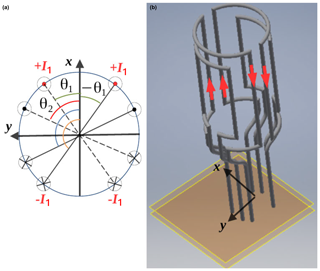Figure 1(a) Electrical conductors of angular positions (θ), flown by currents In, are distributed along the circumference. (b) Illustration of a saddle-like SHS coil with two pairs of coils.

## 3.1 Sensitivity of saddle-like SHS coil

From Biot–Savart law the magnetic field contribution for the four wires of the nth coil can be determined. We assume that the straight conductors forming the coil have a height L. They are located at a distance R to the z axis, at an angular position θn from either side of the x axis and are flown by a current In. The current flowing through these four wires will produce magnetic field contribution (Bwire) in the y-axis direction, given by Eq. (1). The details of the calculation are reported in Appendix A.

$\begin{array}{}\text{(1)}& {\mathrm{Bwire}}_{n}\left(\mathrm{0},\mathrm{0},\mathrm{0}\right)=\frac{{\mathit{\mu }}_{\mathrm{0}}{I}_{n}}{\mathit{\pi }R}\frac{L}{\sqrt{{R}^{\mathrm{2}}+\left(L/\mathrm{2}{\right)}^{\mathrm{2}}}}\mathrm{cos}\left({\mathit{\theta }}_{n}\right)\end{array}$

Next, the magnetic field contribution (at the coil center) of the arc portion (Barcn) in the y-axis direction is given by Eq. (2). The details of the calculation are reported in Appendix A.

$\begin{array}{}\text{(2)}& {\mathrm{Barc}}_{n}\left(\mathrm{0},\mathrm{0},\mathrm{0}\right)=\frac{\mathrm{2}{\mathit{\mu }}_{\mathrm{0}}RL{I}_{n}}{\left({R}^{\mathrm{2}}+\left(L/\mathrm{2}{\right)}^{\mathrm{2}}{\right)}^{\mathrm{3}/\mathrm{2}}}\mathrm{cos}\left({\mathit{\theta }}_{n}\right)\end{array}$

Consequently, the total field at the center $\left({B}_{\mathrm{SHS}}={\mathrm{\Sigma }}_{n=\mathrm{1}}^{N}\left({\mathrm{Bwire}}_{n}\left(\mathrm{0},\mathrm{0},\mathrm{0}\right)+{\mathrm{Barc}}_{n}\left(\mathrm{0},\mathrm{0},\mathrm{0}\right)\right)$ in the y-axis direction) produced by the N pairs of coils of the saddle-like SHS coil will be

$\begin{array}{}\text{(3)}& \begin{array}{rl}{B}_{\mathrm{SHS}}& =\frac{{\mathit{\mu }}_{\mathrm{0}}L}{\sqrt{{R}^{\mathrm{2}}+\left(L/\mathrm{2}{\right)}^{\mathrm{2}}}}\left(\frac{\mathrm{1}}{\mathit{\pi }R}+\frac{\mathrm{2}R}{{R}^{\mathrm{2}}+\left(L/\mathrm{2}{\right)}^{\mathrm{2}}}\right)\\ & {\mathrm{\Sigma }}_{n=\mathrm{1}}^{N}{I}_{n}\mathrm{cos}\left({\mathit{\theta }}_{n}\right).\end{array}\end{array}$

Lastly, by assuming that coils are flown by identical currents ($I={I}_{n}\forall n$), the coil sensitivity (${S}_{\mathrm{coil}}={B}_{\mathrm{SHS}}/I$, cf. ) can be written as

$\begin{array}{}\text{(4)}& \begin{array}{rl}{S}_{\mathrm{coil}}& \propto \frac{{\mathit{\mu }}_{\mathrm{0}}L}{\sqrt{{R}^{\mathrm{2}}+\left(L/\mathrm{2}{\right)}^{\mathrm{2}}}}\left(\frac{\mathrm{1}}{\mathit{\pi }R}+\frac{\mathrm{2}R}{{R}^{\mathrm{2}}+\left(L/\mathrm{2}{\right)}^{\mathrm{2}}}\right)\\ & {\mathrm{\Sigma }}_{n=\mathrm{1}}^{N}\mathrm{cos}\left({\mathit{\theta }}_{n}\right).\end{array}\end{array}$

Consequently, the induced voltage (e(t)), using Hoult formula, is expressed as

$\begin{array}{}\text{(5)}& e\left(t\right)=-{S}_{\mathrm{coil}}\frac{\partial \mathit{\delta }{m}_{xy}\left(t\right)}{\partial t},\end{array}$

where δmxy(t) is the magnetic moment in xy plane and Scoil is the coil sensitivity factor given by Eq. (4). Since the magnetic moment in xy plane precesses at Larmor angular frequency ω0, the induced voltage becomes Eq. (6):

$\begin{array}{}\text{(6)}& \mathrm{|}e\left(j{\mathit{\omega }}_{\mathrm{0}}\right)\mathrm{|}={S}_{\mathrm{coil}}{\mathit{\omega }}_{\mathrm{0}}\mathit{\delta }{m}_{xy}.\end{array}$

## 3.2 Signal-to-noise ratio of saddle-like SHS coil

In this section we will focus on the intrinsic SNR of the SHS coil. We assume round wires and a strong skin effect regime (i.e., skin depth δ much smaller than wire radius); we neglect the proximity effect occurring between closed conductors. Under these approximations, the equivalent copper section of the wire is approximated by Swire=2πrwδ, where rw is the conductor wire radius. On the other side the length of the coil conductor is expressed as ${L}_{\mathrm{coil}}=\mathrm{4}\left(L+R×\left(\mathit{\pi }-\mathrm{2}{\mathit{\theta }}_{n}\right)\right)$. Thus, the electrical resistance of the nth pair of coils (Resn), forming an angle θn, is written as

$\begin{array}{}\text{(7)}& {\mathrm{Res}}_{n}=\frac{\mathrm{2}\mathit{\rho }\left(L+R×\left(\mathit{\pi }-\mathrm{2}{\mathit{\theta }}_{n}\right)\right)}{\mathit{\pi }{r}_{\mathrm{w}}\mathit{\delta }},\end{array}$

where ρ is the conductor material resistivity and $\mathit{\delta }=\sqrt{\mathrm{2}\mathit{\rho }/\mathit{\mu }\mathit{\omega }}$ is the skin depth (μ is the magnetic permeability and ω is the pulsation). Assuming the coils are connected in series, the total resistance (Res) will be the sum of each pair of coil resistance. We then deduced from Eq. (7) the total resistance equation:

$\begin{array}{}\text{(8)}& \mathrm{Res}={\mathrm{\Sigma }}_{n=\mathrm{1}}^{N}Re{s}_{n}=\frac{\mathrm{2}N\mathit{\rho }}{\mathit{\pi }{r}_{\mathrm{w}}\mathit{\delta }}\left(L+R×\left(\mathit{\pi }-\mathrm{2}{\mathrm{\Sigma }}_{n=\mathrm{1}}^{N}{\mathit{\theta }}_{n}/N\right)\right).\end{array}$

Lastly, by assuming a main noise contribution due to Johnson–Nyquist noise (i.e., whose power spectrum density is expressed 4kTRes, where k is the Boltzmann constant and T the temperature in K), the rms voltage noise (vn) of the coil over a frequency range (BW) is deduced from Wiener–Khinchin theorem application ():

$\begin{array}{}\text{(9)}& {v}_{\mathrm{n}}=\sqrt{\mathrm{4}\mathrm{kTRes}×\mathrm{BW}}.\end{array}$

The intrinsic signal-to-noise ratio (SNR) is defined as

$\begin{array}{}\text{(10)}& \mathrm{SNR}=\frac{\mathrm{|}e\left(j{\mathit{\omega }}_{\mathrm{0}}\right)\mathrm{|}}{{v}_{\mathrm{n}}}.\end{array}$

Thus, the SNR of the coil is deduced except for a factor, by combining induced voltage (Eq. 6) and the rms voltage noise (Eq. 9):

$\begin{array}{}\text{(11)}& \mathrm{SNR}\propto \frac{\mathit{\delta }m}{\sqrt{N}}\frac{{\mathrm{\Sigma }}_{n=\mathrm{1}}^{N}\mathrm{cos}\left({\mathit{\theta }}_{n}\right)}{\sqrt{L+R\left(\mathit{\pi }-\mathrm{2}{\mathrm{\Sigma }}_{n=\mathrm{1}}^{N}{\mathit{\theta }}_{n}/N\right)}}.\end{array}$

The SNR improvement can then be appreciated by comparing the SHS4 and SHS6 coils SNR to the one of SHS2 (i.e., the saddle coil). For this purpose, we will consider the angle values for one, two and three pairs of coils obtained by the SHS method () and reiterated here:

• SHS2 (1 pair): ${\mathit{\theta }}_{\mathrm{1}}={\mathrm{30}}^{\circ }$

• SHS4 (2 pairs): ${\mathit{\theta }}_{\mathrm{1}}={\mathrm{12}}^{\circ }$ and ${\mathit{\theta }}_{\mathrm{2}}={\mathrm{48}}^{\circ }$

• SHS6 (3 pairs): ${\mathit{\theta }}_{\mathrm{1}}={\mathrm{11.56}}^{\circ }$, ${\mathit{\theta }}_{\mathrm{2}}={\mathrm{26}}^{\circ }$ and ${\mathit{\theta }}_{\mathrm{3}}={\mathrm{56}}^{\circ }$.

We assume the height-to-radius ratio known to optimize the sensitivity of the saddle coil (i.e., $L=\mathrm{2}R\sqrt{\mathrm{2}}$; see ). In this case, the normalized SNR value estimation (reported in Table 1) shows a significant enhancement for SHS4 and SHS6 with respect to the conventional SHS2 (formal saddle coil).

Table 1Comparison of coil sensitivities obtained by numerical simulation versus coil sensitivities and SNR deduced from the analytic model. The obtained sensitivities and SNR values are normalized with respect to the SHS2 coil.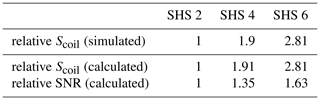4 SHS coils prototypes: designs and experimental results

Various SHS coils are customized in our laboratory, at two extreme frequencies 336 kHz and 128 MHz (corresponding to the 1H Larmor frequency under respectively 8 mT NMR and 3 T MRI) in order to compare their performances in terms of SNR and magnetic field homogeneity (at least for the 3 T MRI case).

## 4.1 SHS coils at low magnetic field (8 mT)

In the context of a low magnetic field NMR dedicated to agronomic studies in planta (), we have designed two SHS coils, referred to as SHS2 and SHS6 (see Fig. 2). The size and geometry of the coils were imposed by the context of the agronomic study: measurements on a sorghum stem. That led us to choose a 30 mm diameter and 40 mm height coil. For practical reasons we used 0.5 mm diameter insulated copper wire as a compromise between the need of high diameter wire (to reduce thermal noise) and the mechanical constraints (flexibility for the winding feasibility). Lastly, the turn number (120 turns, dispatched in three pairs of coils for SHS6) was chosen to get a self-resonance frequency of each coil closed to the 1H Larmor frequency. These coils have the particularity to be clampable in order to manage both the integrity of the plant and the versatility of the NMR instrument.

The measured quality factor of the SHS2 and SHS6 coils were respectively 40 and 80. The SNR of a NMR experiment performed on a same water sample (4 cm3 volume) under the same pulse condition has exhibited a factor of 3 enhancement between the two SHS coils. The details of the testing conditions are reported in . This SNR enhancement, in favor of the SHS6 coil, is slightly higher than the expected one (cf. Table 1). This enhancement could be a consequence of the expected homogeneity improvement since the quantity of magnetization measurable is related to the RF field homogeneity.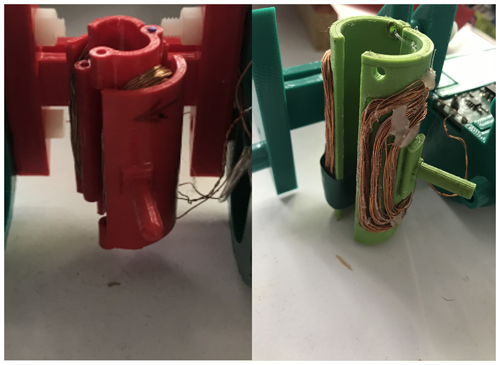Figure 2SHS clampable coils dedicated to the low-field NMR (8 mT) device. Their resonant frequency was tuned closed to 336 kHz (corresponding to the 1H Larmor frequency at 8 mT). Right figure represents the SHS2 clampable coil and left figure the SHS6. The supporting structure has been realized by 3D printing.

## 4.2 SHS coils at high magnetic field (3 T)

### 4.2.1 Experimental setup

A small animal 3 T MRI from RS2D company was used. The 1H resonant frequency into this 3 T MRI is 128 MHz. The reference MRI probe consists in a quadrature decoupled birdcage (from MRI Tools company) whose dimensions are as follows: coil length =80 mm and inner diameter =42 mm. The outer tube diameter is =99 mm in order to guide the antenna into the 100 mm diameter tunnel of the MRI. Birdcage probe and SHS coils used at 3 T were fed through a power splitter device. All the SHS coils were prototyped for the purpose of the study within our lab. The same device was used for both coils.

### 4.2.2 SHS4 coil 3 T prototype

At higher frequency, MRI probe designer must take care that the coil length remains smaller than a fraction of the wavelength ($\sim \mathrm{1}/\mathrm{10}$) at the frequency of interest. When this is not the case, the MRI coil must be segmented () to ensure that the quasi-static magnetic field hypothesis is suitable. For the high field condition (i.e., implying high frequency operating), the coil wire of the SHS coil must be segmented as illustrated in Fig. 3.

.Figure 3Electrical circuit of the segmented SHS4 coil (3 T prototype) with tuning–matching circuit: CS is a fixed segmentation capacitance, CT is a variable capacitance, CpreM is a fixed matching capacitance and CM is a variable capacitance allowing to match finely the impedance of the coil near the Larmor frequency

Finally, a quadrature-driven SHS4 coil prototype (illustrated in Fig. 4), dedicated to 1H measuring in a small-animal 3 T MRI, has been constructed. Its dimensions were chosen identical to the ones of the reference birdcage given above. The coil itself is realized on a flexible printed circuit board with a copper ribbon width equal to 3 mm. The flexible circuit includes pads to welcome the segmentation capacitors. To allow the quadrature driving, two identical flexible circuits were used. Each flexible circuit was associated with its own tuning and matching circuit (whose configuration circuit is similar to the one presented in ). The segmented capacitance values of the prototypes are experimentally determined to coincide with the electrical resonance and Larmor frequencies. For the SHS 6 prototype CS=44 pF was chosen and the coil was divided into 4 segments of length ∼25 cm in order to equalize with electrical resonance frequency to the Larmor one. CT, CM and CpreM are respectively the tuning, matching and “pre-matching capacitances. CT and CM are non-magnetic variable capacitances from VOLTRONICS, while CS and CpreM are non-magnetic fixed capacitances from TEMEX.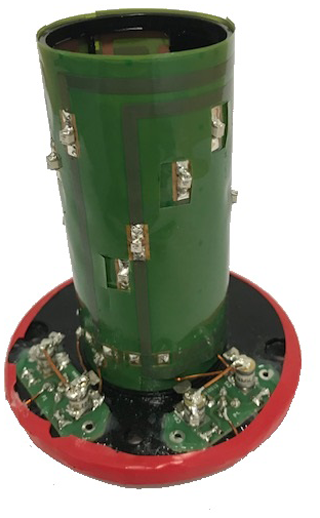Figure 4Quadrature SHS4 serial coil dedicated to 1H 3 T MRI (80 mm length and 42 mm internal diameter). The coils are realized on a same flexible printed circuit board including the pads to welcome the segmentation capacitors. Two tuning–matching PCB, for respectively 0 and 90 driving, are inserted on the lower part of the coil.

### 4.2.3 A comparison: SHS4 coil versus birdcage

Images have been acquired using the 3 T MRI from RS2D company using a spin echo sequence. The sample consisted in a cylindrical tube (26 mm diameter – 38 mm length) filled of water + CuS04 (allowing a decrease in the longitudinal relaxation time). The images obtained using a 12-leg quadrature birdcage coil on one hand and quadrature SHS4 coil on the other hand are reported in Fig. 5. Probes were fed through a 090 hybrid coupler device. For testing in single-channel mode the hybrid coupler was used through the 0 channel, while a 50Ω was put at the output of the 0 channel.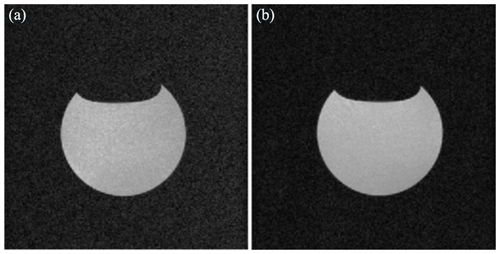Figure 5(a) is the one obtained using the quadrature birdcage while (b) has been obtained using the quadrature SHS4 coil. The imaging sequence is a MRI spin echo sequence with following parameters: TE=10 ms (echo time), TR=600 ms (repetition time), field of view $=\mathrm{50}\phantom{\rule{0.125em}{0ex}}\mathrm{mm}×\mathrm{50}\phantom{\rule{0.125em}{0ex}}\mathrm{mm}$, slice thickness =1 mm, average =1 and resolution $=\mathrm{256}×\mathrm{256}$).

Next, the SNR measurements between different 1H 3 T coils (i.e., birdcage coil, saddle coil and SHS4 coil, single channel or quadrature-driven) are summarized in Table 2.

Table 2SNR comparisons between birdcage coil, saddle coil and SHS4 measured on the same sample, with identical pulse sequence parameters, in a 3 T MRI from RS2D; n/a denotes not applicable.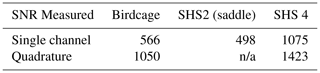5 Discussion

The experimental SNR comparison of SHS prototypes at low and high magnetic field with respect to both saddle coil and birdcage coil validated the modeled SNR increase. This SNR increase was also confirmed between SHS4 and birdcage using quadrature-driven coil (∼35 %). However, it is noticeable that the single- and quadrature-channel mode exhibit a SNR difference higher than the classical 3 dB. We hypothesize this difference is attributable to this non-optimal configuration. The homogeneity of quadrature SHS4 coil proven by image acquiring is roughly comparable to the one of birdcage (also in quadrature), making the saddle-like SHS coil a relevant MRI probe competitor. However, the superiority of SHS coil could be limited for higher-volume samples in which the noise will become dominated by magnetic and dielectric noise. From Eq. (11) we can roughly express the SNR dependency as $\propto \frac{{\mathrm{\Sigma }}_{n=\mathrm{1}}^{N}\mathrm{cos}\left({\mathit{\theta }}_{n}\right)}{\sqrt{N}}$; thus, for instance using four pairs of coils, the SNR of the SHS coil would increase by approximately a factor of 2. However, the increased of number of pairs of coils could be limited by complexity and especially at high frequency where the occurrence of the proximity effect becomes preponderant.

6 Conclusions

The SHS method allows one to easily determine the position of electrical conductor favoring the production of a homogenous magnetic field. The method was previously tested in the context of spherical coil for static magnetic field. The present work concerned the design of NMR or MRI probes based on this method. Saddle-like SHS coils have been constructed for different frequency regimes (from 336 kHz up to 128 MHz) in the context of 1H NMR and MRI. The saddle-like SHS coil has been proved to be driven in quadrature. The experimental comparisons between saddle-like SHS coil, saddle coil and commercial birdcage of comparable sizes validate the higher performances in terms of both homogeneity and SNR of the SHS coils. The higher performance obtained makes the SHS probe valuable for NMR and MRI experiment. Additionally, the great simplicity of design of SHS coils, with respect to the complexity of the birdcage, should be highlighted.

Appendix A: Detailed calculation of Bwiren and Barcn

We consider first a single wire “1” of height L parallel to z and centered in the xy plane whose middle is located at a distance R from the orthonormal, forming an angle θ with x axis, while α corresponds to the angle between the position vector (r) and the xy plane. The magnetic field generated by this “1” wire at the center of the SHS coil is expressed using Biot–Savart law:

$\begin{array}{}\text{(A1)}& \mathrm{d}\mathbit{B}=\frac{{\mathit{\mu }}_{\mathrm{0}}I}{\mathrm{4}\mathit{\pi }}\frac{\mathrm{d}\mathbit{l}\wedge \mathbit{r}}{\parallel \mathbit{r}{\parallel }^{\mathrm{3}}},\end{array}$

where $\mathrm{d}\mathbit{l}=\left[\mathrm{0};\mathrm{0};\mathrm{d}z\right]$, $\mathbit{r}=\left[R\mathrm{cos}\left(\mathit{\theta }\right);-R\mathrm{sin}\left(\mathit{\theta }\right);z\right]$, z=Rtan α and $\parallel \mathbit{r}\parallel =\sqrt{{R}^{\mathrm{2}}+{z}^{\mathrm{2}}}$, which becomes $\parallel \mathbit{r}\parallel =R/\mathrm{cos}\left(\mathit{\alpha }\right)$. Thus dz is expressed $R/{\mathrm{cos}}^{\mathrm{2}}\mathit{\alpha }×\mathrm{d}\mathit{\alpha }$. It results that dB has only components in the xy plane:

$\mathrm{d}\mathbit{B}=\frac{{\mathit{\mu }}_{\mathrm{0}}I}{\mathrm{4}\mathit{\pi }\parallel \mathbit{r}{\parallel }^{\mathrm{3}}}\left[\begin{array}{c}Rdz\mathrm{sin}\left(\mathit{\theta }\right)\\ Rdz\mathrm{cos}\left(\mathit{\theta }\right)\\ \mathrm{0}\end{array}\right].$

Then,

$\mathrm{d}\mathbit{B}=\frac{{\mathit{\mu }}_{\mathrm{0}}I}{\mathrm{4}\mathit{\pi }R}\mathrm{cos}\left(\mathit{\alpha }\right)\mathrm{d}\mathit{\alpha }\left[\begin{array}{c}\mathrm{sin}\left(\mathit{\theta }\right)\\ \mathrm{cos}\left(\mathit{\theta }\right)\\ \mathrm{0}\end{array}\right].$

The integration of dB over the wire length (from $-L/\mathrm{2}$ to $+L/\mathrm{2}$) is expressed

$\begin{array}{c}\mathrm{d}\mathbit{B}=\underset{-\mathrm{arctan}\left(L/\left(\mathrm{2}R\right)\right)}{\overset{\mathrm{arctan}\left(L/\left(\mathrm{2}R\right)\right)}{\int }}\frac{{\mathit{\mu }}_{\mathrm{0}}I}{\mathrm{4}\mathit{\pi }R}\mathrm{cos}\left(\mathit{\alpha }\right)\mathrm{d}\mathit{\alpha }\left[\begin{array}{c}\mathrm{sin}\left(\mathit{\theta }\right)\\ \mathrm{cos}\left(\mathit{\theta }\right)\\ \mathrm{0}\end{array}\right]\\ \mathbit{B}=\frac{{\mathit{\mu }}_{\mathrm{0}}I}{\mathrm{2}\mathit{\pi }R}\mathrm{sin}\left(\mathrm{arctan}\left(L/\left(\mathrm{2}R\right)\right)\right)\left[\begin{array}{c}\mathrm{sin}\left(\mathit{\theta }\right)\\ \mathrm{cos}\left(\mathit{\theta }\right)\\ \mathrm{0}\end{array}\right].\end{array}$

Let us now calculate sin (arctan (u)) where we define θ=arctan (u). First, we start from $\mathrm{tan}\left(\mathit{\theta }\right)=\mathrm{sin}\left(\mathit{\theta }\right)/\mathrm{cos}\left(\mathit{\theta }\right)$, which can be rewritten

$\begin{array}{}\text{(A2)}& \mathrm{tan}\left(\mathrm{arctan}\left(u\right)\right)=\frac{\mathrm{sin}\left(\mathrm{arctan}\left(u\right)\right)}{\mathrm{cos}\left(\mathrm{arctan}\left(u\right)\right)},\end{array}$

which becomes

$\begin{array}{}\text{(A3)}& \mathrm{sin}\left(\mathrm{arctan}\left(u\right)\right)=u×\mathrm{cos}\left(\mathrm{arctan}\left(u\right)\right).\end{array}$

Next, since

$\begin{array}{}\text{(A4)}& \mathrm{1}+{\mathrm{tan}}^{\mathrm{2}}\left(\mathit{\theta }\right)=\mathrm{1}/{\mathrm{cos}}^{\mathrm{2}}\left(\mathit{\theta }\right),\end{array}$

we get

$\begin{array}{}\text{(A5)}& \mathrm{1}+{\mathrm{tan}}^{\mathrm{2}}\left(\mathrm{arctan}\left(u\right)\right)=\mathrm{1}/{\mathrm{cos}}^{\mathrm{2}}\left(\mathrm{arctan}\left(u\right)\right),\end{array}$

which becomes

$\begin{array}{}\text{(A6)}& \mathrm{cos}\left(\mathrm{arctan}\left(u\right)\right)=\frac{\mathrm{1}}{\sqrt{\mathrm{1}+{u}^{\mathrm{2}}}}.\end{array}$

By combining these equations we finally get

$\begin{array}{}\text{(A7)}& \mathrm{sin}\left(\mathrm{arctan}\left(u\right)\right)=\frac{u}{\sqrt{\mathrm{1}+{u}^{\mathrm{2}}}}.\end{array}$

Thanks to the writing of sin (arctan (u)) we express the magnetic field:

$\mathbit{B}=\frac{{\mathit{\mu }}_{\mathrm{0}}I}{\mathrm{4}\mathit{\pi }R}\frac{L}{\sqrt{{R}^{\mathrm{2}}+\left(L/\mathrm{2}{\right)}^{\mathrm{2}}}}\left[\begin{array}{c}\mathrm{sin}\left(\mathit{\theta }\right)\\ \mathrm{cos}\left(\mathit{\theta }\right)\\ \mathrm{0}\end{array}\right].$

Lastly, we sum the contribution of the four wires. By writing ${B}_{w}=\frac{{\mathit{\mu }}_{\mathrm{0}}I}{\mathrm{4}\mathit{\pi }R}\frac{L}{\sqrt{{R}^{\mathrm{2}}+\left(L/\mathrm{2}{\right)}^{\mathrm{2}}}}$, each magnetic field contribution for wires 1−4 is expressed

$\begin{array}{}\text{(A8)}& {\mathbit{B}}_{\mathrm{1}}={B}_{\mathrm{w}}\left[\mathrm{sin}\left(\mathit{\theta }\right);\mathrm{cos}\left(\mathit{\theta }\right);\mathrm{0}\right]\text{(A9)}& {\mathbit{B}}_{\mathrm{2}}={B}_{\mathrm{w}}\left[-\mathrm{sin}\left(\mathit{\theta }\right);\mathrm{cos}\left(\mathit{\theta }\right);\mathrm{0}\right]\text{(A10)}& {\mathbit{B}}_{\mathrm{3}}={B}_{\mathrm{w}}\left[-\mathrm{sin}\left(\mathit{\theta }\right);\mathrm{cos}\left(\mathit{\theta }\right);\mathrm{0}\right]\text{(A11)}& {\mathbit{B}}_{\mathrm{4}}={B}_{\mathrm{w}}\left[\mathrm{sin}\left(\mathit{\theta }\right);\mathrm{cos}\left(\mathit{\theta }\right);\mathrm{0}\right].\end{array}$

Thus the resultant magnetic field of the wires portion for nth coil (Bwiren), at an angular position θn and flown by a current In, will have contribution in y-axis direction which is given by

$\begin{array}{}\text{(A12)}& {\mathrm{Bwire}}_{n}=\frac{{\mathit{\mu }}_{\mathrm{0}}{I}_{n}}{\mathit{\pi }R}\frac{L}{\sqrt{{R}^{\mathrm{2}}+\left(L/\mathrm{2}{\right)}^{\mathrm{2}}}}cos\left({\mathit{\theta }}_{n}\right).\end{array}$

Next, the contribution of an arc portion (in the xy plane, thus z=0) is obtained by writing the elementary circuit portion: $\mathrm{d}\mathbit{l}=\left[R\mathrm{d}\mathit{\theta }\mathrm{cos}\left(\mathit{\theta }-\mathit{\pi }/\mathrm{2}\right);R\mathrm{d}\mathit{\theta }\mathrm{sin}\left(\mathit{\theta }-\mathit{\pi }/\mathrm{2}\right);\mathrm{0}\right]$ and the distance vector $\mathbit{r}=\left[R\mathrm{cos}\left(\mathit{\theta }\right);R\mathrm{sin}\left(\mathit{\theta }\right);z\right]$. Since two arc portions are opposite, the opposite elementary circuit portion is $\mathrm{d}{\mathbit{l}}^{\mathbf{\prime }}=\left[R\mathrm{d}\mathit{\theta }\mathrm{cos}\left(\mathit{\pi }/\mathrm{2}-\mathit{\theta }\right);R\mathrm{d}\mathit{\theta }\mathrm{sin}\left(\mathit{\pi }/\mathrm{2}-\mathit{\theta }\right);\mathrm{0}\right]$. The resulting contribution of d$\mathbit{l}+\mathrm{d}{\mathbit{l}}^{\mathbf{\prime }}$ produces a magnetic field contribution (dBarc1) given by

$\mathrm{d}{\mathbit{B}}_{\mathrm{arc}\mathrm{1}}=\frac{{\mathit{\mu }}_{\mathrm{0}}I}{\left({R}^{\mathrm{2}}+{z}^{\mathrm{2}}{\right)}^{\mathrm{3}/\mathrm{2}\right)}}\left[\begin{array}{c}-\mathrm{2}Rz\mathrm{d}\mathit{\theta }\mathrm{cos}\left(\mathit{\theta }-\mathit{\pi }/\mathrm{2}\right)\\ \mathrm{2}{R}^{\mathrm{2}}\mathrm{d}\mathit{\theta }\mathrm{sin}\left(\mathit{\theta }\right)\mathrm{cos}\left(\mathit{\theta }-\mathit{\pi }/\mathrm{2}\right)\\ \mathrm{0}\end{array}\right].$

Then, the same contribution produced by the two opposite arc but shifted at $z=L/\mathrm{2}$ becomes

$\mathrm{d}{\mathbit{B}}_{\mathrm{arc}\mathrm{1}}={\mathit{\alpha }}_{\mathrm{1}}\left[\begin{array}{c}-\mathrm{2}R\left(z-L/\mathrm{2}\right)\mathrm{d}\mathit{\theta }\mathrm{cos}\left(\mathit{\theta }-\mathit{\pi }/\mathrm{2}\right)\\ \mathrm{2}{R}^{\mathrm{2}}\mathrm{d}\mathit{\theta }\mathrm{sin}\left(\mathit{\theta }\right)\mathrm{cos}\left(\mathit{\theta }-\mathit{\pi }/\mathrm{2}\right)\\ \mathrm{0}\\ ,\end{array}\right]$

where ${\mathit{\alpha }}_{\mathrm{1}}=\frac{{\mathit{\mu }}_{\mathrm{0}}I}{\left({R}^{\mathrm{2}}+\left(z-L/\mathrm{2}{\right)}^{\mathrm{2}}{\right)}^{\mathrm{3}/\mathrm{2}\right)}}$. Similarly, the contribution of the two arcs at position $z=-L/\mathrm{2}$ and flown by current I is written as

$\mathrm{d}{\mathbit{B}}_{\mathrm{arc}\mathrm{2}}={\mathit{\alpha }}_{\mathrm{2}}\left[\begin{array}{c}-\mathrm{2}R\left(z+L/\mathrm{2}\right)\mathrm{d}\mathit{\theta }\mathrm{cos}\left(\mathit{\theta }-\mathit{\pi }/\mathrm{2}\right)\\ \mathrm{2}{R}^{\mathrm{2}}\mathrm{d}\mathit{\theta }\mathrm{sin}\left(\mathit{\theta }\right)\mathrm{cos}\left(\mathit{\theta }-\mathit{\pi }/\mathrm{2}\right)\\ \mathrm{0}\end{array}\right],$

where ${\mathit{\alpha }}_{\mathrm{2}}=-\frac{{\mathit{\mu }}_{\mathrm{0}}I}{\left({R}^{\mathrm{2}}+\left(z+L/\mathrm{2}{\right)}^{\mathrm{2}}{\right)}^{\mathrm{3}/\mathrm{2}\right)}}$. The combination of dBarc2 and dBarc2 at the center of the coil (z=0) is written as

$\mathrm{d}{\mathbit{B}}_{\mathrm{arc}}=\frac{{\mathit{\mu }}_{\mathrm{0}}I}{\left({R}^{\mathrm{2}}+\left(L/\mathrm{2}{\right)}^{\mathrm{2}}{\right)}^{\mathrm{3}/\mathrm{2}\right)}}\left[\begin{array}{c}\mathrm{2}RL\mathrm{d}\mathit{\theta }\mathrm{cos}\left(\mathit{\theta }-\mathit{\pi }/\mathrm{2}\right)\\ \mathrm{0}\\ \mathrm{0}\end{array}\right].$

Lastly, the integration of dBarc over θ will be

$\begin{array}{}\text{(A13)}& {\mathrm{dBarc}}_{n}=\frac{\mathrm{2}{\mathit{\mu }}_{\mathrm{0}}RL{I}_{n}}{\left({R}^{\mathrm{2}}+\left(L/\mathrm{2}{\right)}^{\mathrm{2}}{\right)}^{\mathrm{3}/\mathrm{2}}}\mathrm{cos}\left({\mathit{\theta }}_{n}\right).\end{array}$

By integration over θn, the total contribution on y axis of the arc portion at the center of the coil (Barcn) is given by

$\begin{array}{}\text{(A14)}& {\mathrm{Barc}}_{n}=\frac{\mathrm{2}{\mathit{\mu }}_{\mathrm{0}}RL{I}_{n}}{\left({R}^{\mathrm{2}}+\left(L/\mathrm{2}{\right)}^{\mathrm{2}}{\right)}^{\mathrm{3}/\mathrm{2}}}\mathrm{cos}\left({\mathit{\theta }}_{n}\right).\end{array}$
Data availability
Data availability.

All the required data to reproduce the experiment are given in the body of the paper (geometric parameters of the MRI coils, the electrical component values, and the parameters of the MRI pulse sequence).

Author contributions
Author contributions.

PdP was responsible for the high magnetic field (9.4 and 3 T) SHS MRI coil design and testing and contributed to writing the paper. LP carried out the high magnetic field (9.4 and 3 T) SHS MRI coil design and testing. RSB carried out the low magnetic field (8 mT) SHS coil design and testing and also contributed to writing the paper. MZ carried out the SHS conceptualization and modeling. EN carried out the SHS conceptualization and modeling. EA was responsible for the mechanical design of the 3 T coils. SR was responsible for the mechanical design of the 8 mT coils. MC managed the high magnetic field MRI tests. JLV, together with NB, obtained the clampable MRI coil solution for plants, and they were responsible of funding and supervision of each of the two projects (grant no. 1504-005 and Pari scientifique INRA). CGB is the head of the MRI facility at Montpellier University. JM managed 3 T MRI testing and comparisons. RS is the head of the 3 T MRI facility at RS2D. CC carried out the SHS conceptualization, modeling, MRI coil tests, and manuscript writing, reviewing, and editing.

Competing interests
Competing interests.

The authors declare that they have no conflict of interest.

Acknowledgements
Acknowledgements.

The authors would like to thank SATT AxLR (who funded the prototyping program), CNRS (who funded in 2018 the so-called “Défi instrumentation aux limites”), Agropolis fondation in the context of APLIM (Advanced Plant Life Imaging) project (contract 1504-005) and Pari scientifique INRA département Environnement et Agronomie.

Financial support
Financial support.

This research has been supported by the SATT AxLR, the Centre National de la Recherche Scientifique (Défi Instrumentation aux Limites), the Agropolis Fondation (grant no. 1504-005), and the INRA (Pari scientifique INRA département Environnement et Agronomie).

Review statement
Review statement.

This paper was edited by Marco Jose da Silva and reviewed by two anonymous referees.

References

Bloch, F.: Nuclear Induction, Phys. Rev., 70, 460–474, 1946. a

Bolinger, L., Prammer, M. G., and Leigh, J. S.: A Multiple-Frequency Coil with a Highly Uniform B1 Field, J. Magn. Reson., 81, 162–166, 1988. a

Clark, J. W.: A new method for obtaining a uniform magnetic field, Rev. Sci. Instrum., 9, 320–322, 1938. a

Coillot, C., Nativel, E., Zanca, M., and Goze-Bac, C.: The magnetic field homogeneity of coils by means of the space harmonics suppression of the current density distribution, J. Sens. Sens. Syst., 5, 401–408, https://doi.org/10.5194/jsss-5-401-2016, 2016a. a, b

Coillot, C., Sidiboulenouar, R., Nativel, E., Zanca, M., Alibert, E., Cardoso, M., Saintmartin, G., Noristani, H., Lonjon, N., Lecorre, M., Perrin, F., and Goze-Bac, C.: Signal modeling of an MRI ribbon solenoid coil dedicated to spinal cord injury investigations, J. Sens. Sens. Syst., 5, 137–145, https://doi.org/10.5194/jsss-5-137-2016, 2016b. a

Hayes, C. E., Edeutein, W. A., Schenck, J. F., Mueller, O. M., and Eash, M.: An Efficient Highly Homogeneous Radiofrequency Coil for Whole-Body NMR Imaging at 1.5T, J. Magn. Reson., 63, 622–628, 1985. a

Hoult, D. I. and Richards, R. E.: The signal-to-noise ratio of the nuclear magnetic resonance experiment, J. Magn. Reson., 24, 71–85, 1976. a, b

Khintchine, A.: Math. Ann., 109, 604–615, https://doi.org/10.1007/BF01449156, 1934. a

Mispelter, J., Lupu, M., and Briguet, A.: NMR probehads for biophysical and biomedical experiments: theoretical principles and practical guidelines, Imperial College Press, 2006. a, b, c

Sidi-Boulenouar, R.: Dynamic monitoring of water status of plants in the fields under environmental stress: Design of a portable NMR and applied to sorghum, Physics [physics], Université Montpellier, available at: https://tel.archives-ouvertes.fr/tel-02139254/document (last access: 17 March 2020), 2018. a

Sidi-Boulenouar, R., Reis, A., Nativel, E., Buy, S., de Pellegars, P., Liu, P., Zanca, M., Goze-Bac, C., Barbat, J., Alibert, E., Verdeil, J.-L., Gatineau, F., Bertin, N., Anand, A., and Coillot, C.: Homogenous static magnetic field coils dedicated to portable nuclear magnetic resonance for agronomic studies, J. Sens. Sens. Syst., 7, 227–234, https://doi.org/10.5194/jsss-7-227-2018, 2018. a, b